Department of Computer Science & Mathematics

Topology Research Group

Nipissing University
 MEMBERS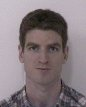Logan Hoehn Assistant professor Research interests: General and Geometric Topology (continuum theory, logic and set theory), Dynamical Systems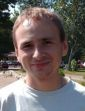Alex Karassev Associate professor Research interests: General and Geometric Topology (dimension theory, asymptotic topology, infinite-dimensional topology, continuous selections, continuum theory), Functional Analysis (algebraic properties of spaces of functions, ranks of C*-algebras) Ihor Stasyuk Postdoctoral fellow Research interests: General and Geometric Topology (function spaces, metric spaces, selections, extension operators)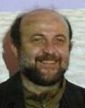Murat Tuncali Professor Research interests: General and Geometric Topology (dimension theory, continuum theory), Dynamical Systems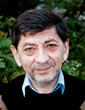Vesko Valov Professor Research interests: General and Geometric Topology (dimension theory, infinite-dimensional topology, continuous selections, homogeneous spaces), Functional Analysis (algebraic properties of spaces of functions, ranks of C*-algebras, spaces of measures)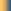Group HomeTopology Seminar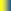Group MembersPopular LecturesNUMERICFields InstituteNSERCTopology AtlasArxiv.orgThe Geometry Center